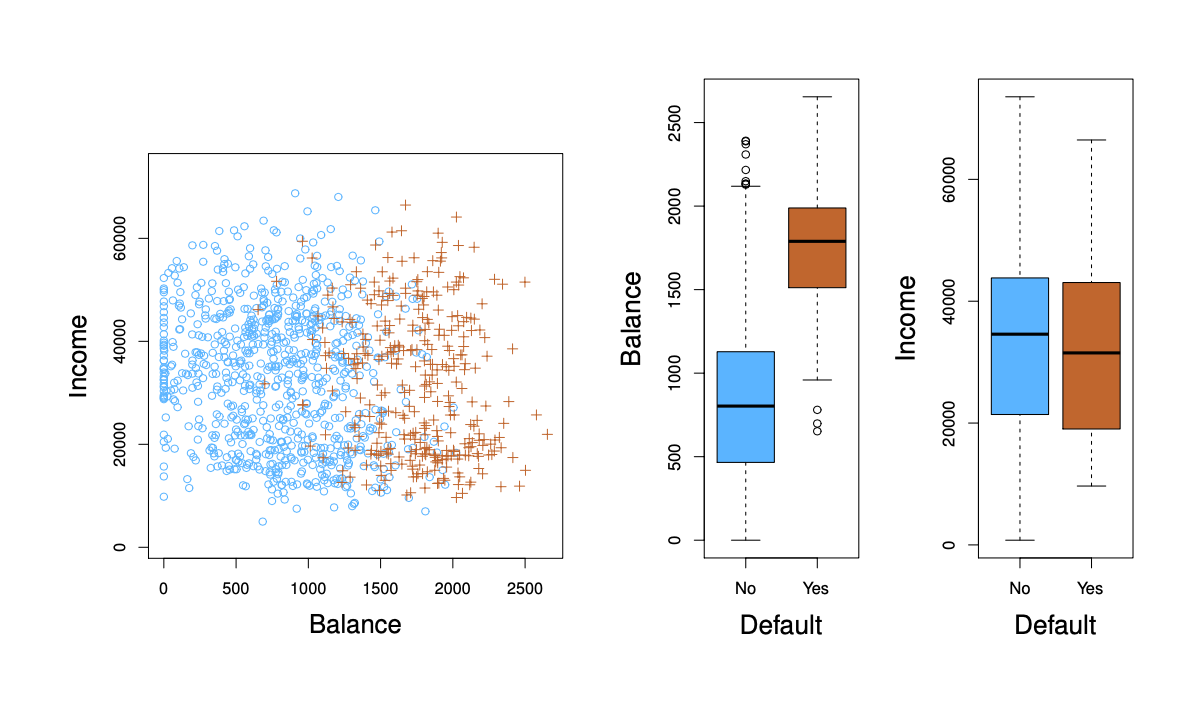## Introduction to Classification

• Recall, for linear regression the response variable $$Y$$ is quantitative and generally thought of as continuous

• How do we handle response variables that are qualitative (i.e., categorical)?

• For example, eye color
• Predicting a qualitative response for an observation can be referred to as classifying that observation, since it involves assigning the observation to a category, or class

• Often the methods used for classification first predict the probability that the observation belongs to each of the categories of a qualitative variable, as the basis for making the classification

• Widely used classifiers:

• Logistic Regression, Linear Discriminant Analysis, quadratic Discriminant Analysis, Naive Bayes, and K-Nearest Neighbors

## Classification

• Qualitative variables take values in an unordered set $$C$$

• eye color $$\in$$ {brown, blue, green}

• color $$\in$$ {blue, red, green, yellow}

• Given a feature vector $$X$$ and a qualitative response $$Y$$ taking values in the set $$C$$, the classification task is to build a function $$C(X)$$ that takes as a input the feature vector $$X$$ and predicts its value for $$Y$$

• Often we are more interested in estimating the probabilities that $$X$$ belongs to each category in $$C$$

• For example, it is more valuable to have an estimate of the probability that an insurance claim is fraudulent, than a classification fraudulent or not• We are interested in predicting whether an individual will default on his or her credit card payment, on the basis of annual income and monthly credit card balance

• In the left-hand panel, we have plotted annual income and monthly credit card balance for a subset of 10,000 individuals

• The individuals who defaulted in a given month are shown in orange, and those who did not in blue
• In the center and right-hand panels, two pairs of boxplots are shown. The first shows the distribution of balance split by the binary default variable; the second is a similar plot for income

## Can We Use Linear Regression?

• Suppose for the example above that we code the response as follows:

• $$Y = \left\{\begin{matrix} 0 \\ 1 \end{matrix}\right.$$

• Where 0 equals “No”

• And 1 equals “Yes”

• Can we simply perform linear regression of Y on X and classify as $$Yes$$ if $$\hat{Y}$$ > 0.5

• In this case of a binary outcome, linear regression does a decent job as a classifier and is equivalent to Linear Discriminant Analysis (discussed below)

• Since in the population $$E(Y|X = x)= Pr(Y = 1 | X = X)$$, we might think that regression is perfect for this task

• However, linear regression might produce probabilities that are less than zero or larger than 1

• Logistic regression is more appropriate